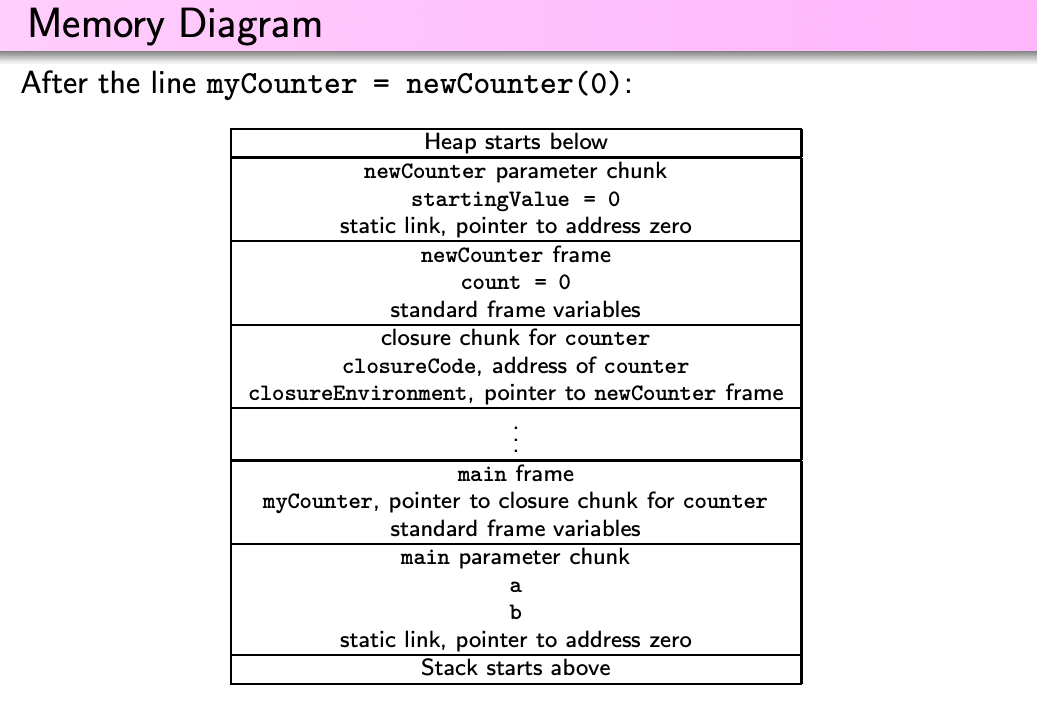# Closure §

A closure is a pair of a function pointer (address of the code of the body of the function) and an environment (mapping free variables to their values).

We use closures to implement Function Values.

There are two operations associated with closures:

1. Closure Creation: Creates a closure value from a procedure,
2. Closure Call: Invokes the function represented by a closure value.
def increaseByLacs ( increment : Int ): ( Int )=> Int = {
def procedure (x : Int ) = x + increment
procedure // closure creation
}
increase = increaseByLacs(3)
increase(5) // closure call

Closures are these things inside another function, and so the closure environment in this case is increaseByLacs.

In LACS, when we do an assignment, increase is going to create a new typed variable?

when you just use ID, that is a closure creation (like when you return procedure inside increaseByLacs). And when you put it around brackets, then it becomes

factor -> factor LPAREN argsopt RPAREN


so factor can be an ID, or it can be another factor lparen argsopt rparen

in which case you can treat it as a procedure?

Think of a closure as a procedure and a binding of free variables to values.

### Implementing Closures §

This is really hard and complicated.

if a procedure is made into a closure, then the frames and parameter chunks of all of its outer procedures must be allocated on the heap.

To implement a closure creation, we must compute the two addresses that go into the new closure:

1. Function pointer is straightforward: it is the code address (label) of the procedure from which we are creating the closure (the procedure called procedure in our example), and this procedure is specified at the closure creation site.
2. Environment: address of the frame of the procedure directly enclosing the procedure from which we are creating the closure. It turns out that this is the same address that we would pass as the static link if we were directly calling the procedure instead of creating a closure out of it. Therefore, the environment for the closure is computed in exactly the same way as we would compute the static link for a call to the same procedure.

To implement a closure call, we need to make use of the two addresses in the closure.

• The code address tells us where the code of the function is; this is the address that we load into the program counter using the JALR instruction.
• The environment provides values for free variables of the function value. Since the code of the function accesses these free variables using its static link, it follows that we must pass the environment address retrieved from the closure as the static link for the function that we call.

The issue is that the extent of these variables go beyond a simple Stack, the environment can be modified after it’s created, so we need to use a Heap.

def counter():Int = {
count = count + 1;
count
}
// assume count is bound to 0
var newCounter:()=>Int;
newCounter = counter;
newCounter(); // returns 1
newCounter(); // returns 2

Consider the following program

def main(a:Int,b:Int):Int = {
var myCounter:()=>Int;
myCounter = newCounter(0); // stores a closure chunk in myCounter
myCounter(); // Closure call, returns 1
myCounter(); // Closure Call, returns 2
}
def newCounter(startingValue:Int):()=>Int = {
var count:Int;
def counter():Int = { count = count + 1; count }
count = startingValue;
counter // Closure Creation
}

Here’s the memory diagram of the aboveAlso see Procedure for these memory diagrams.

### Python §

https://realpython.com/python-lambda/#python-lambda-and-regular-functions

I was running into this issue https://www.reddit.com/r/manim/comments/p1w619/list_of_updater_functions_issue/

I really don’t understand how this works.